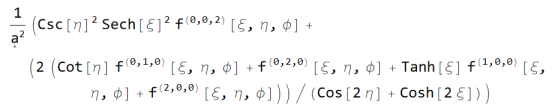# Laplacian in various coordinate systems

The recent post on the wave equation on a disk showed that the Laplace operator has a different form in polar coordinates than it does in Cartesian coordinates. In general, the Laplacian is not simply the sum of the second derivatives with respect to each variable.

Mathematica has a function, unsurprisingly called Laplacian, that will compute the Laplacian of a given function in a given coordinate system. If you give it a specific function, it will compute the Laplacian of that function. But you can also give it a general function to find a general formula.

For example,

    Simplify[Laplacian[f[r, θ], {r, θ}, "Polar"]]

returnsThis is not immediately recognizable as the Laplacian from this postbecause Mathematica is using multi-index notation, which is a little cumbersome for simple cases, but much easier to use than classical notation when things get more complicated. The superscript (0,2), for example, means do not differentiate with respect to the first variable and differentiate twice with respect to the second variable. In other words, take the second derivative with respect to θ.

Here’s a more complicated example with oblate spheroidal coordinates. Such coordinates come in handy when you need to account for the fact that our planet is not exactly spherical but is more like an oblate spheroid.

    Simplify[Laplacian[f[ξ, η, φ], {ξ, η, φ}, "OblateSpheroidal"]]

the result isn’t pretty.I tried using TeXForm and editing it into something readable, but after spending too much time on this I gave up and took a screenshot. But as ugly as the output is, it would be uglier (and error prone) to do by hand.

Mathematica supports the following 12 coordinate systems in addition to Cartesian coordinates:

• Cylindrical
• Bipolar cylindrical
• Elliptic cylindrical
• Parabolic cylindrical
• Circular parabolic
• Confocal paraboloidal
• Spherical
• Bispherical
• Oblate spheroidal
• Prolate spheroidal
• Conical
• Toroidal

These are all orthogonal, meaning that surfaces where one variable is held constant meet at right angles. Most curvilinear coordinate systems used in practice are orthogonal because this simplifies a lot of things.

Laplace’s equation is separable in Stäckel coordinate systems. These are all these coordinate systems except for toroidal coordinates. And in fact Stäckel coordinates are the only coordinate systems in which Laplace’s equation is separable.

It’s often the case that Laplace’s equation is separable in orthogonal coordinate systems, but not always. I don’t have a good explanation for why toroidal coordinates are an exception.

If you’d like a reference for this sort of thing, Wolfram Neutsch’s tome Coordinates is encyclopedic. However, it’s expensive new and hard to find used.